Lion data set as 2-way ANOVA

#The following sets up the data fro the analysis
#Set working directory

setwd("C:/Users/lisanjie2/Desktop/TEACHING/1_STATS_CalU/1_STAT_CalU_2016_by_NLB/Lecture/Unit3_regression/last_week")

Lion data

plot(age.years ~ portion.black, data = dat,
main = "Lion pigment data split into 3 groups",
ylim = c(0,19))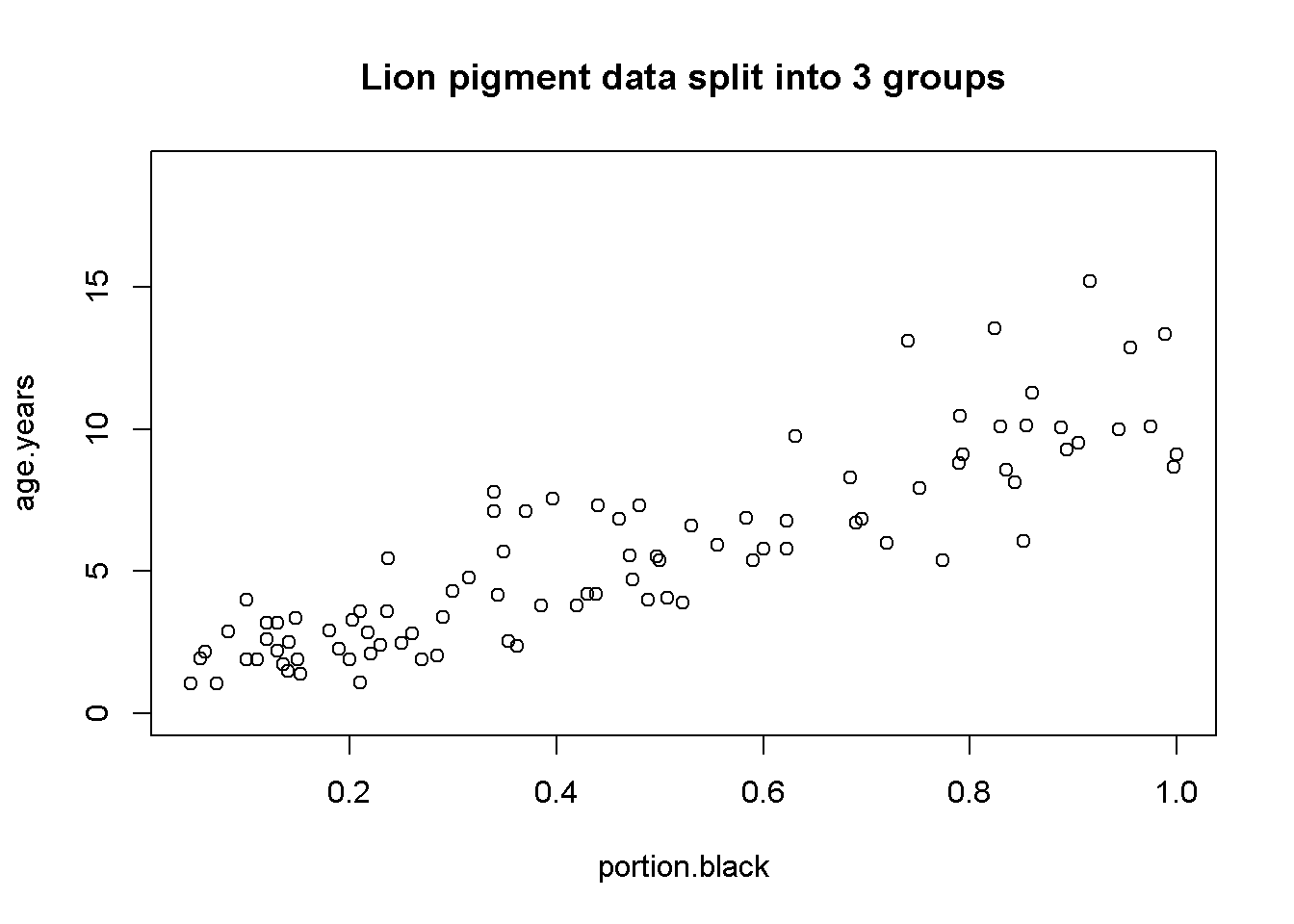Split lion data by groups

par(mfrow = c(1,1))

dat$pigment.groups.2 <- "high.pigment" dat$pigment.groups.2[which(dat$portion.black <= pigment.mean)] <- "low.pigment" dat$pigment.groups.2 <- factor(dat$pigment.groups.2) summary(dat) ## age.years portion.black population sex ## Min. : 1.056 Min. :0.04609 Ngorogoro:10 female:61 ## 1st Qu.: 2.800 1st Qu.:0.21000 Serengeti:83 male :32 ## Median : 5.378 Median :0.43000 ## Mean : 5.589 Mean :0.46446 ## 3rd Qu.: 7.800 3rd Qu.:0.72000 ## Max. :15.178 Max. :1.00000 ## pigment.groups.2 ## high.pigment:43 ## low.pigment :50 ## ## ## ## dat$pigment.groups.2 <- factor(dat$pigment.groups.2, levels =c("low.pigment", "high.pigment")) table(dat$pigment.groups.2, dat$population) ## ## Ngorogoro Serengeti ## low.pigment 6 44 ## high.pigment 4 39 table(dat$pigment.groups.2, dat$sex) ## ## female male ## low.pigment 25 25 ## high.pigment 36 7 NOTE: Splitting up a continuous variable like this into a categorical variable and doing an ANOVA is generally a BAD idea. Regression is the proper way to analyze the raw data. I am making these groups for illustration purposes only! Plot raw data by pigmentation group par(mfrow = c(1,1)) plot(age.years ~ pigment.groups.2,data = dat, xlab = "Pigmentation group", ylab = "Known Lion Age")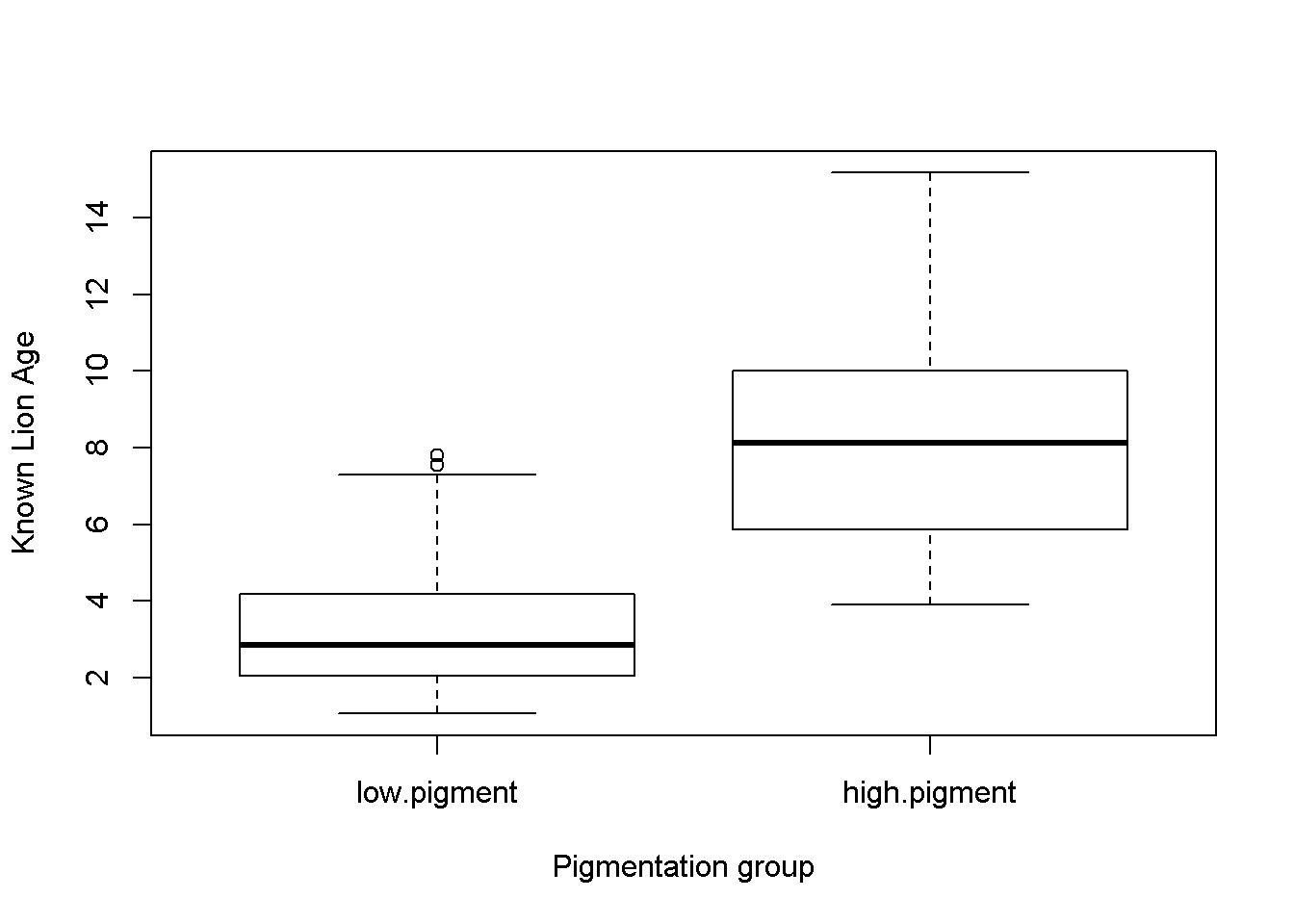Figure 1a: Boxplots of lion ages in two pigmentation groups from the Serengeti and Ngorogoro Crater, Tanzania, east Africa. Plot raw data by pigmentation group par(mfrow = c(1,1)) plot(age.years ~ population,data = dat, xlab = "Pigmentation group", ylab = "Known Lion Age")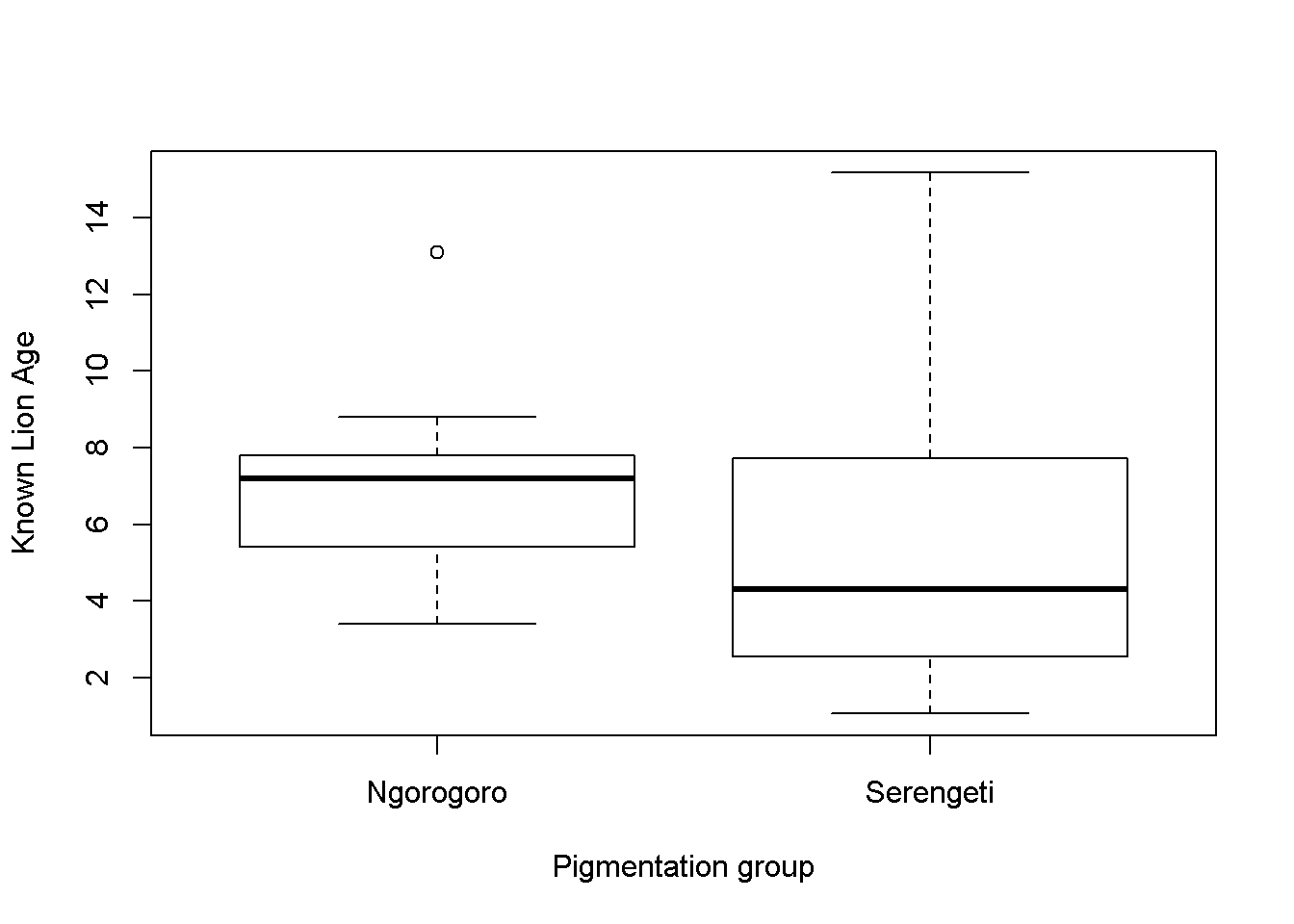Figure 1b: Boxplots of lion ages in two populations the Serengeti and Ngorogoro Crater, Tanzania, east Africa. Plot means library(sciplot) ci.fun. <- function(x) c(mean(x)-2*se(x), mean(x)+3*se(x)) lineplot.CI(x.factor = pigment.groups.2, response = age.years, data = dat, ci.fun = ci.fun. )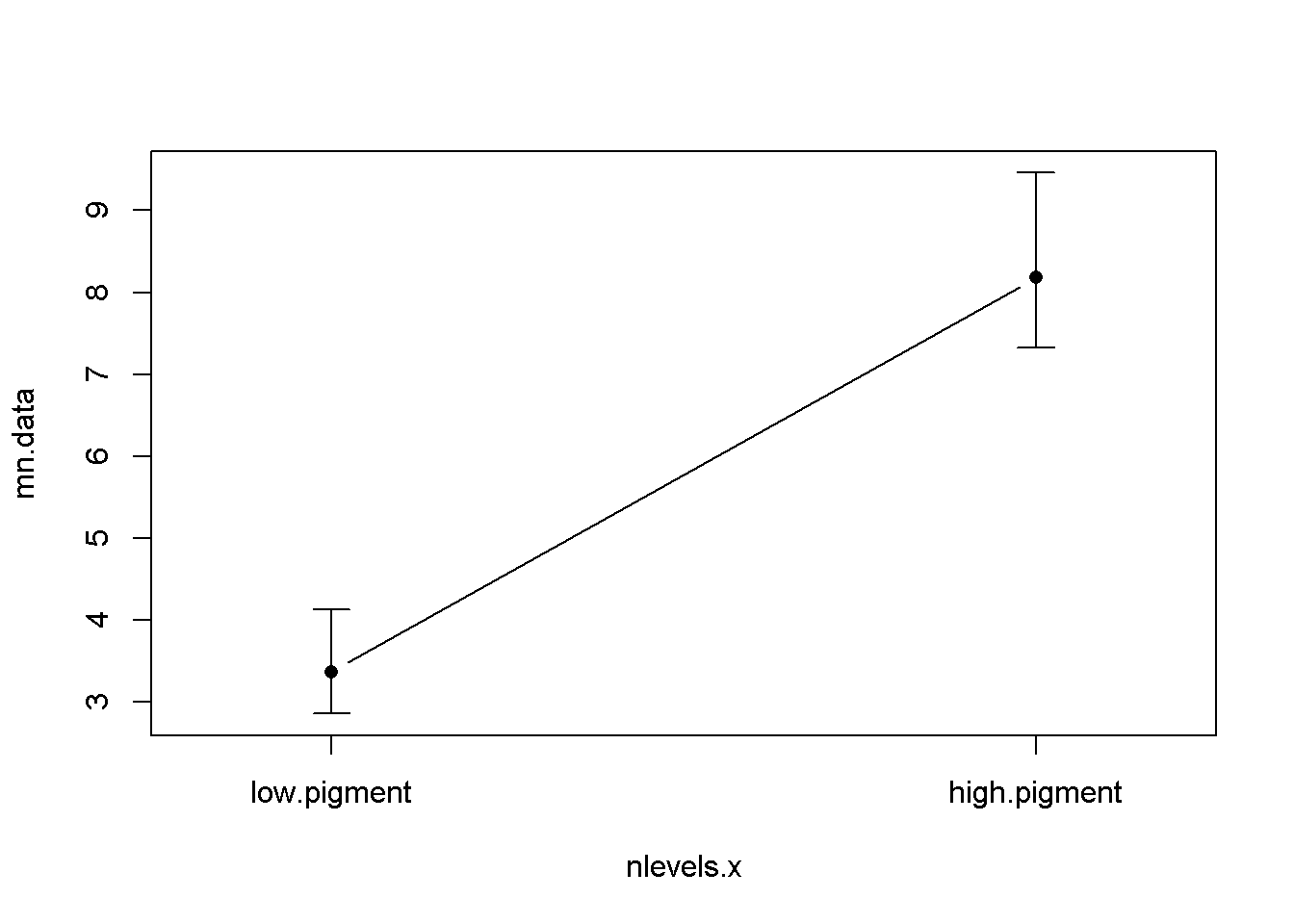Figure 2a: Mean age of lions in two pigmentation groups from the Serengeti and Ngorogoro crater, Tanzania, east Africa. Error bars are approximate 95% confidence intervals. lineplot.CI(x.factor = population, response = age.years, data = dat, ci.fun = ci.fun. )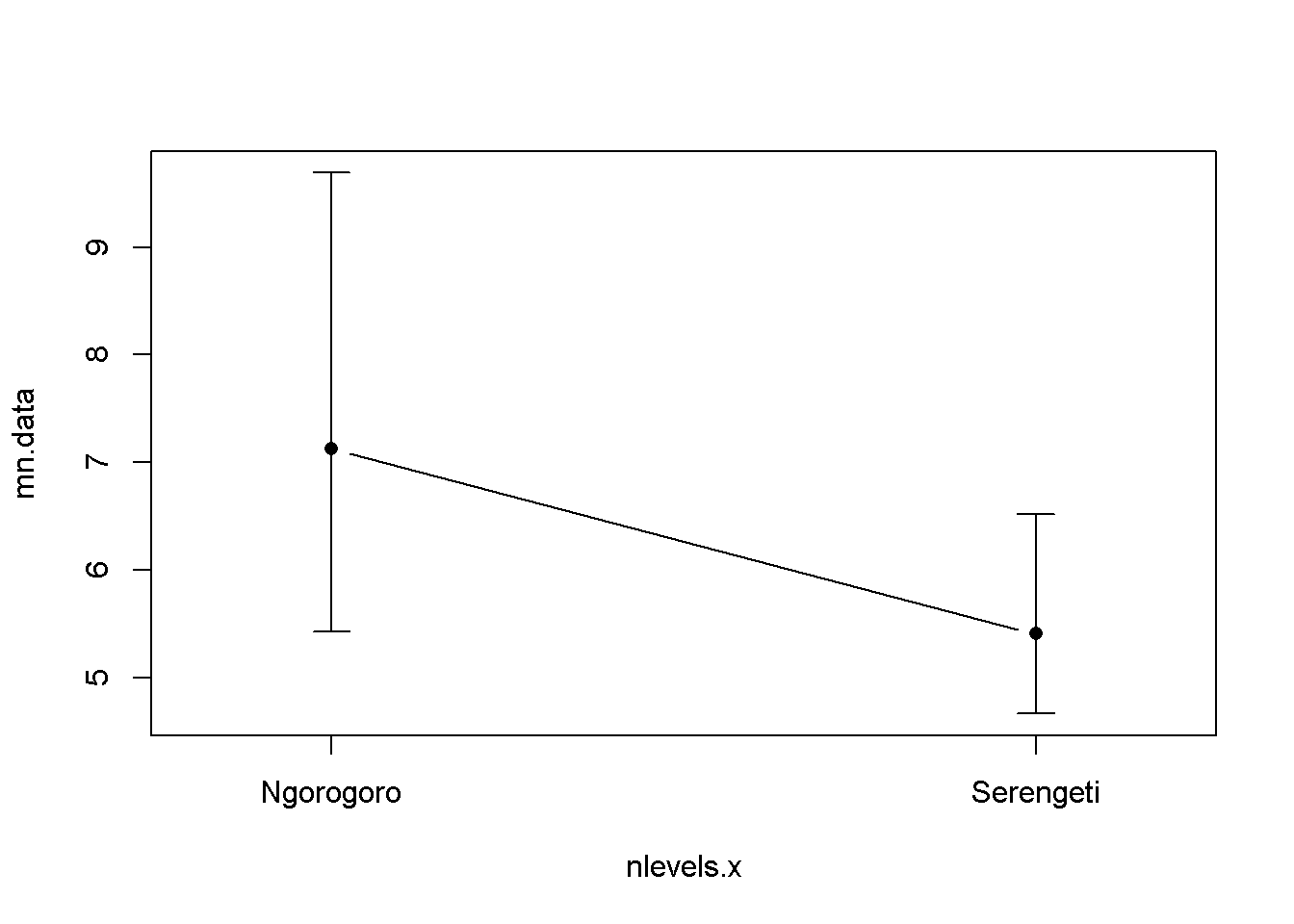Figure 2b: Mean age of lions in two population groups, the Serengeti and Ngorogoro crater, Tanzania, east Africa. Error bars are approximate 95% confidence intervals. Calcualte the means of each of the FOUR groups source("plot_means.R") #combine group names dat$all.four.groups <- paste(dat$population, dat$pigment.groups.2)

means <- tapply(dat$age.years, dat$all.four.groups,
mean)

sds <- tapply(dat$age.years, dat$all.four.groups,
sd)

ns <- tapply(dat$age.years, dat$all.four.groups,
length)

summary(factor(dat$all.four.groups)) ## Ngorogoro high.pigment Ngorogoro low.pigment Serengeti high.pigment ## 4 6 39 ## Serengeti low.pigment ## 44 groups <- c("Ngorogoro high", "Ngorogoro low", "Serengeti high", "Serengeti low") ses <- sds/sqrt(ns) plot.means(means = means, SEs = ses, categories = groups,y.axis.label = "Lion Age")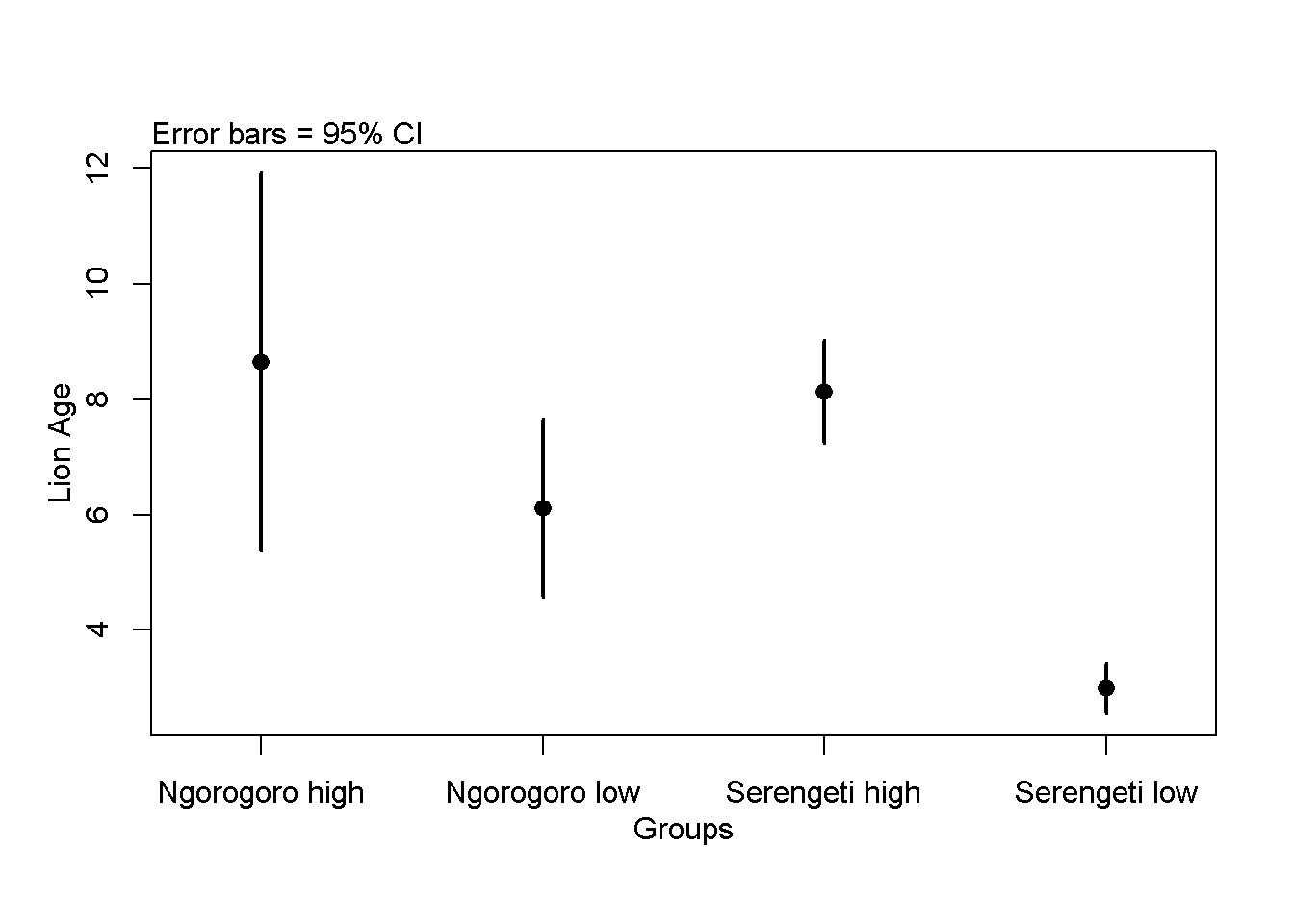Two-way ANOVA (again, don’t do an analysis like this - use the original data and do an ANOVA!) Null model: age.years ~ 1 Age does not vary with pigmentation OR pigmentation m.null <- lm(age.years ~ 1 ,data = dat) Alternative model 1: age.years ~ pigment.groups.2 Hypoth:Age does vary with pigmentation m.alt.1.pigment <- lm(age.years ~ pigment.groups.2,data = dat) Alternative model 2: age.years ~ population Hypoth:Age varies with population m.alt.2.pop <- lm(age.years ~ population,data = dat) Alternative model 3: age.years ~ pigmentation + population Hypoth:Age varies with population AND pigmentation m.alt.3.both <- lm(age.years ~ population +pigment.groups.2,data = dat) Alternative model 4: age.years ~ pigmentation *population (FUll model) Hypoth:Age varies with population m.alt.4.intxn <- lm(age.years ~ population*pigment.groups.2,data = dat) m.alt.4.mean <- lm(age.years ~ -1+ population:pigment.groups.2,data = dat) THe hard way: Omnibus ANOVA F-test on EACH set of models Null vs. alt.1 (pigmentation groups) anova(m.null, m.alt.1.pigment) ## Analysis of Variance Table ## ## Model 1: age.years ~ 1 ## Model 2: age.years ~ pigment.groups.2 ## Res.Df RSS Df Sum of Sq F Pr(>F) ## 1 92 1025.42 ## 2 91 489.14 1 536.28 99.77 2.697e-16 *** ## --- ## Signif. codes: 0 '***' 0.001 '**' 0.01 '*' 0.05 '.' 0.1 ' ' 1 Null vs. alt.3 (populations) anova(m.alt.1.pigment, m.alt.2.pop) ## Analysis of Variance Table ## ## Model 1: age.years ~ pigment.groups.2 ## Model 2: age.years ~ population ## Res.Df RSS Df Sum of Sq F Pr(>F) ## 1 91 489.14 ## 2 91 998.83 0 -509.69 Null vs. alt.3 (pigmentation + population) anova(m.alt.1.pigment, m.alt.3.both) ## Analysis of Variance Table ## ## Model 1: age.years ~ pigment.groups.2 ## Model 2: age.years ~ population + pigment.groups.2 ## Res.Df RSS Df Sum of Sq F Pr(>F) ## 1 91 489.14 ## 2 90 451.09 1 38.048 7.5912 0.007098 ** ## --- ## Signif. codes: 0 '***' 0.001 '**' 0.01 '*' 0.05 '.' 0.1 ' ' 1 Null vs. alt.4 (pigmentation*population interaction) anova(m.alt.3.both, m.alt.4.intxn) ## Analysis of Variance Table ## ## Model 1: age.years ~ population + pigment.groups.2 ## Model 2: age.years ~ population * pigment.groups.2 ## Res.Df RSS Df Sum of Sq F Pr(>F) ## 1 90 451.09 ## 2 89 436.44 1 14.652 2.9878 0.08736 . ## --- ## Signif. codes: 0 '***' 0.001 '**' 0.01 '*' 0.05 '.' 0.1 ' ' 1 The easy way anova() on “interaction” model anova(m.alt.4.intxn) ## Analysis of Variance Table ## ## Response: age.years ## Df Sum Sq Mean Sq F value Pr(>F) ## population 1 26.60 26.60 5.4234 0.02213 * ## pigment.groups.2 1 547.74 547.74 111.6958 < 2e-16 *** ## population:pigment.groups.2 1 14.65 14.65 2.9878 0.08736 . ## Residuals 89 436.44 4.90 ## --- ## Signif. codes: 0 '***' 0.001 '**' 0.01 '*' 0.05 '.' 0.1 ' ' 1 Writing up result of Omnibus F-test Summarize reulst of ANOVA in a single sentence: “There was a significant difference in the mean ages of the three diffrent pigmentation groups (F = 106.67, p < 0.00001, DF = 92,90)” Comparisons using Tukey-HSD Refit m.alt w/ aov() dat$pigment.groups.2.rename <- gsub("pigment","",dat\$pigment.groups.2)

m.alt.4.inxtn.aov <- aov(age.years ~ pigment.groups.2.rename*population,data = dat)

Pairwise comparison w/TukeyHSD()

Run TukeyHSD()

my.tukey.intxn <- TukeyHSD(m.alt.4.inxtn.aov)